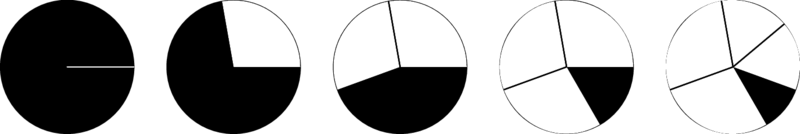# Internet Problem Solving Contest

## Problem F – Flipping and cutting

On the table we have a perfect circle cut out of paper. The circle is all black from the top and all white from the bottom. The circumference of the circle is a positive integer c.

We also have a pair of scissors. We will use the scissors to cut the circle. At the beginning we will choose an arbitrary point on the boundary of the circle (we’ll call it the active point) and we’ll make a straight cut from that point to the center of the circle.

Next, we will choose a positive integer s (with s < c2): the square of our step length. Finally, we are going to perform an infinite sequence of rounds. Each round consists of a few simple steps:

1. Find a new point by starting at the current active point and measuring the distance $\sqrt{s}$ along the boundary of the circle, going counter-clockwise.
2. Make a straight cut from the new point to the center of the circle.
3. We now have a wedge (a sector of the circle) that starts with the cut from the active point to the center and continues counter-clockwise all the way to the cut from the new point to the center. Note that the inside of the wedge may also contain other cuts.
4. We carefully lift the entire wedge from the table, flip it upside down and return it back to its place. Note that if the wedge already consisted of multiple pieces, their order is reversed by this operation.
5. The new point becomes the active point for the next round.

The figure below illustrates the first four rounds for c = 360 and $\sqrt{s}=100$. Pay close attention to what happens in the fourth round.### Problem specification

For some pairs (c, s) it may happen that after finitely many rounds we will have a completely black circle again.

Find out which pairs (c, s) have this property, and for each such pair determine the smallest number of rounds after which it happens.

(A technical note: A circle is completely black if each point of the top side of the circle is black or lies on one of the cuts. In other words, you don’t have to worry about the color of the points that lie directly on the cuts.)

### Input specification

The first line of the input file contains an integer t specifying the number of test cases. Each test case is preceded by a blank line.

Each test case consists of a single line containing the positive integers c and s. As stated above, you may assume that s < c2.

In the easy subproblem F1 you may also assume that c ≤ 1 000 and that $\sqrt{s}$ is an integer.

In the hard subproblem F2 you may assume that c ≤ 106.

### Output specification

If the process never returns to a completely black circle, output a single line with the text “never”. Otherwise, output a single line with a single integer: the smallest positive number of rounds after which the circle will again be completely black.

### Example

Input:

3

360 3600

360 10000

360 90000

Output:

12
24
4

In the first example case we have a circle with circumference 360 units and we are cutting wedges that each contain 60 units of the circle’s circumference. Clearly, after six rounds we will have flipped each part of the circle exactly once, and after another six rounds everything will be back to where we started.

The second example input corresponds to the situation shown in the figure above. It takes 24 rounds to get us back to an all-black circle.

In the third example we flip 5/6 of the circle in each round. Maybe surprisingly, already after four rounds the circle will be completely black again.